Previous Page | Next Page

 The MCMC Procedure

## Example 52.9 Normal Regression with Interval Censoring

You can use PROC MCMC to fit failure time data that can be right, left, or interval censored. To illustrate, a normal regression model is used in this example.

Assume that you have the following simple regression model with no covariates: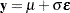where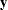is a vector of response values (the failure times),is the grand mean,
is an unknown scale parameter, andare errors from the standard normal distribution. Instead of observing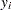directly, you only observe a truncated value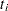. If the trueoccurs after the censored time, it is called right censoring. Ifoccurs before the censored time, it is called left censoring. A failure timecan be censored at both ends, and this is called interval censoring. The likelihood foris as follows: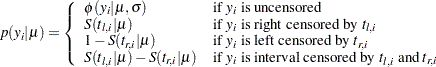where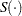is the survival function,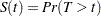.

Gentleman and Geyer (1994) uses the following data on cosmetic deterioration for early breast cancer patients treated with radiotherapy:

```title 'Normal Regression with Interval Censoring';
data cosmetic;
label tl = 'Time to Event (Months)';
input tl tr @@;
datalines;
45  .   6 10   .  7  46  .  46  .   7 16  17  .   7 14
37 44   .  8   4 11  15  .  11 15  22  .  46  .  46  .
25 37  46  .  26 40  46  .  27 34  36 44  46  .  36 48
37  .  40  .  17 25  46  .  11 18  38  .   5 12  37  .
.  5  18  .  24  .  36  .   5 11  19 35  17 25  24  .
32  .  33  .  19 26  37  .  34  .  36  .
;
```

The data consist of time interval endpoints (in months). Nonmissing equal endpoints (tl = tr) indicates uncensoring; a nonmissing lower endpoint (tl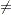.) and a missing upper endpoint (tr = .) indicates right censoring; a missing lower endpoint (tl = .) and a nonmissing upper endpoint (tr.) indicates left censoring; and nonmissing unequal endpoints (tltr) indicates interval censoring.

With this data set, you can consider using proper but diffuse priors on bothand, for example: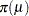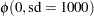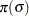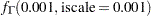where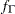is the gamma density function.

The following SAS statements fit an interval censoring model and generate Output 52.9.1:

```proc mcmc data=cosmetic outpost=postout seed=1 nmc=20000 missing=AC;
ods select PostSummaries PostIntervals;
parms mu 60 sigma 50;

prior mu ~ normal(0, sd=1000);
prior sigma ~ gamma(shape=0.001,iscale=0.001);

if (tl^=. and tr^=. and tl=tr) then
llike = logpdf('normal',tr,mu,sigma);
else if (tl^=. and tr=.) then
llike = logsdf('normal',tl,mu,sigma);
else if (tl=. and tr^=.) then
llike = logcdf('normal',tr,mu,sigma);
else
llike = log(sdf('normal',tl,mu,sigma) -
sdf('normal',tr,mu,sigma));

model general(llike);
run;
```

Because there are missing cells in the input data, you want to use the MISSING=AC option so that PROC MCMC does not delete any observations that contain missing values. The IF-ELSE statements distinguish different censoring cases for, according to the likelihood. The SAS functions LOGCDF, LOGSDF, LOGPDF, and SDF are useful here. The MODEL statement assigns llike as the log likelihood to the response. The Markov chain appears to have converged in this example (evidence not shown here), and the posterior estimates are shown in Output 52.9.1.

Output 52.9.1 Interval Censoring
 Normal Regression with Interval Censoring

The MCMC Procedure

Posterior Summaries
Parameter N Mean Standard
Deviation
Percentiles
25% 50% 75%
mu 20000 41.7807 5.7882 37.7220 41.3468 45.2249
sigma 20000 29.1122 6.0503 24.8774 28.2210 32.4250

Posterior Intervals
Parameter Alpha Equal-Tail Interval HPD Interval
mu 0.050 32.0499 54.6104 31.3604 53.6115
sigma 0.050 20.0889 43.1335 19.4041 41.6742Previous Page | Next Page | Top of Page